Author Message
SnarlingSheep
Professional Member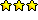Joined: 13 Mar 2001
Posts: 759
Location: MichiganPosted: Tue Sep 06, 2005 6:08 pm    Post subject: Convert Numbers to Words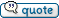This is a quick example of converting something like 599.09 to "Five Hundred Ninety Nine Dollars and Nine Cents."
Updated to work up to 999 Trillion... can easily go on from there.
 Code: OPTION decimalsep,"." REM *** Number to convert %%number = @input(Enter number:,"6,278,567,909,520.33") list create,1 list loadtext,1 "One "Two "Three "Four "Five "Six "Seven "Eight "Nine "Ten list create,2 list loadtext,2 "Eleven "Twelve "Thirteen "Fourteen "Fifteen "Sixteen "Seventeen "Eighteen "Nineteen list create,3 list loadtext,3 "Twenty "Thirty "Fourty "Fifty "Sixty "Seventy "Eighty "Ninety REM Remove Commas repeat   %%comma = @POS(",",%%number)   if @GREATER(%%comma,0)     %%number = @strdel(%%number,%%comma,%%comma)   end until @EQUAL(%%comma,0) %%number = @format(%%number,0.2) REM *** Split dollars and cents if needed %%decimal = @pos(".",%%number) if %%decimal   option fieldsep,"."   parse "%%number;%%cents", %%number end %%stop = 0 %%thousands = 0   repeat     %%processnumber = @substr(%%number,@succ(@fsub(@len(%%number),3)),@len(%%number))     GOSUB Process3Digit     if @equal(%%thousands,0)       %%text = %%currtext     end     if @equal(%%thousands,1)       %%text = %%currtext Thousand"," %%text     end     if @equal(%%thousands,2)       %%text = %%currtext Million"," %%text     end    if @equal(%%thousands,3)       %%text = %%currtext Billion"," %%text     end    if @equal(%%thousands,4)       %%text = %%currtext Trillion"," %%text     end    REM If number is more than 3 digits, cut off the last 3     if @greater(@len(%%number),3)       %%number = @strdel(%%number,@succ(@fsub(@len(%%number),3)),@len(%%number))    else    REM If not, stop the loop      %%stop = 1     end     %%thousands = @succ(%%thousands)   until @equal(%%stop,1) REM *** Process Cents if @greater(%%cents,0)   if @not(@equal(@substr(%%cents,1,1),0))     if @not(@equal(@substr(%%cents,2,2),0))       if @equal(@substr(%%cents,1,1),1)        %%centstext = @item(2,@pred(@substr(%%cents,2,2))) Cents        else         %%centstext = @item(3,@fsub(@substr(%%cents,1,1),2)) @item(1,@pred(@substr(%%cents,2,2))) Cents      end    else      %%centstext = @item(1,@pred(@substr(%%cents,1,1))) Cents    end   else     %%centstext = @item(1,@pred(@substr(%%cents,2,2))) Cents   end end REM Show Results if @greater(@len(%%text),0)   %%text = %%text Dollars and end  info @trim(%%text %%centstext) exit :Process3Digit   REM *** If number is one digit, get it's name from list 1   if @equal(@len(%%processnumber),1)     %%currtext = @item(1,@pred(%%processnumber))   end   REM *** If number is two digits and the first digit is 1, get name from list 2   if @equal(@len(%%processnumber),2)     if @equal(@substr(%%processnumber,1,1),1)       %%currtext = @item(2,@pred(@substr(%%processnumber,2,2)))     else       %%currtext = @item(3,@fsub(@substr(%%processnumber,1,1),2)) @item(1,@pred(@substr(%%processnumber,2,2)))     end   end   if @equal(@len(%%processnumber),3)     %%currtext = @item(1,@pred(@substr(%%processnumber,1,1))) Hundred @item(3,@fsub(@substr(%%processnumber,2,2),2)) @item(1,@pred(@substr(%%processnumber,3,3)))   end   exit

_________________
-Sheep
My pockets hurt...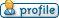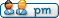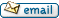Display posts from previous: All Posts1 Day7 Days2 Weeks1 Month3 Months6 Months1 Year Oldest FirstNewest First
 All times are GMT Page 1 of 1